## 20141101

### Physics presentation: elasticity"Unbowed, Unbent, Unbroken." Uh, yeah, looks like all three were contradicted here.In this presentation we will only be discussing the elastic behavior of materials--what will happen when they stretched or squished non-destructively ("bowed"), and can still return to their original state afterwards. As for "bent" and "broken," we'll defer that discussion as our focus will be on avoiding permanent distortion or damage to materials.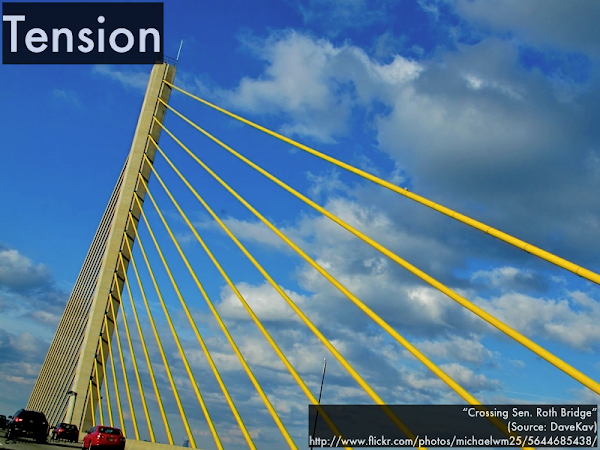Tension is when you stretch something, like these bridge suspension cables.Compression is when you squish something, like the stones near the bottom of this stack.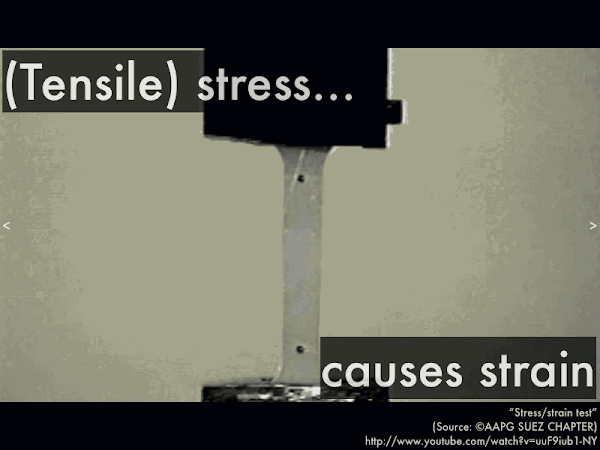More precisely, tensile stress is applying a force to a certain cross-section sample to stretch it, while tensile strain is a measure of how the material responds, (although here, the sample does not return to its original state, stretched permanently beyond its elastic limits). (Video link: "Stress/strain test.")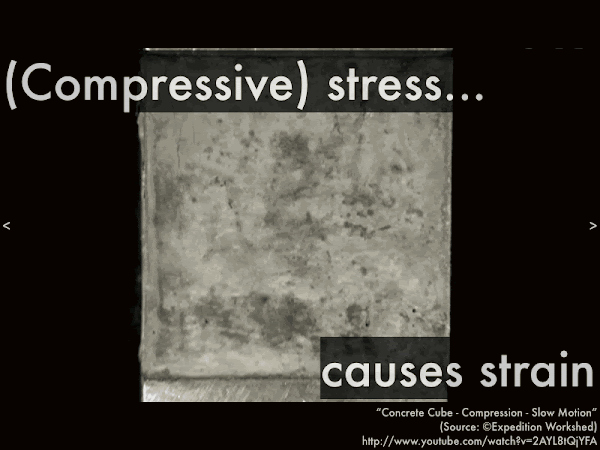And as expected, compressive stress is applying a force to a certain cross-section sample to squish it, while compressive strain is a measure of how the material responds, (although here, the sample does not return to its original state, after cracking and crumbling). (Video link: "Concrete Cube - Compression - Slow Motion.")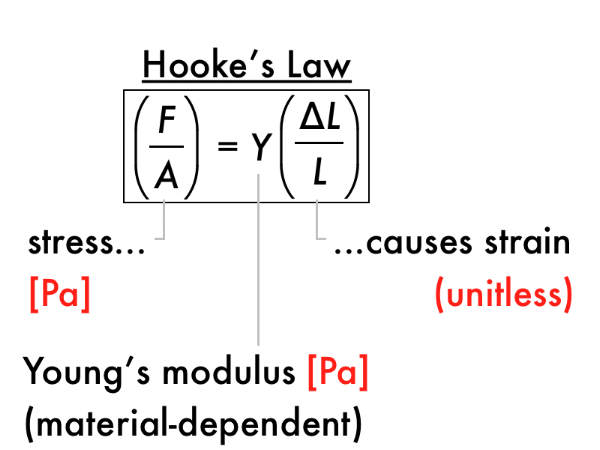Hooke's law is the quantitative relation between tensile/compressive stress (force exerted per unit area, in N/m2, or Pa), and strain (given as a fractional length change, unitless), with a material-dependent Young's modulus (in units of Pa) that characterizes the response of the material to these stresses.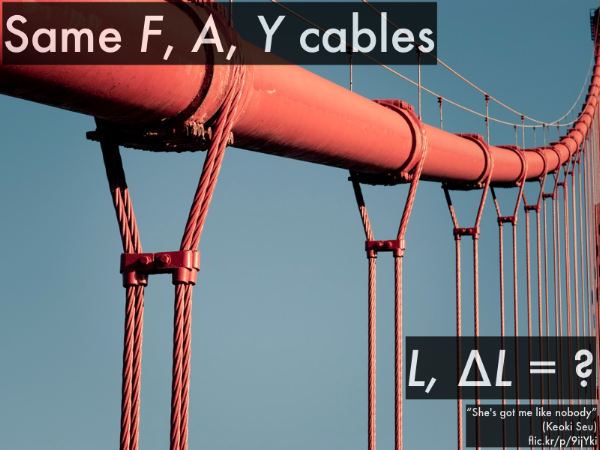For these vertical suspension bridge cables, they all have the same cross-sectional area A and Young's modulus Y (being made of the same material). Assume that each cable has the same amount of tension F, as they are evenly spaced and presumably carry the weight of equal sections of the bridge.

For the different lengths of cable, which are stretched by the least amount (say, measured in cm) from their original lengths: the shorter lengths of cable, or the longer lengths of cable?

Let's rewrite Hooke's law in terms of ∆L and L, as these are different for the short and long lengths of cable, and have cross-sectional area A, Young's modulus Y, and the tension F all on the other side of the equation, as these quantities are all the same for both short and long lengths of cable:

(∆L/L) = F/(A·Y).

So the right-hand side of this equation is the same for both the short and long lengths of cable:

(∆Lshort/Lshort) = F/(A·Y),

(∆Llong/Llong) = F/(A·Y),

so we can set the left-hand sides of both these equations equal to each other:

(∆Lshort/Lshort) = (∆Llong/Llong).

Since Lshort < Llong, then for the equality to hold, ∆Lshort < ∆Llong, and thus the shorter cable will stretch by a smaller amount than the longer cable.For these long 2×4 boards used as bracing, note that some are two 2×4s columns while others are three 2×4 columns, effectively having different cross-sectional areas. However, they all have the same Young's modulus Y (being made of the same material), and the same length L. Assume that each column experiences the same amount of ∆L compression from their original length (such that they all have the same amount of strain).

For the different cross-sectional area columns, which support the least amount of force: the narrower cross-sectional area columns, or the wider cross-sectional area columns?